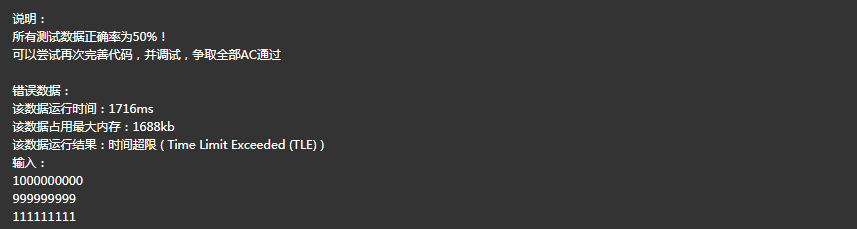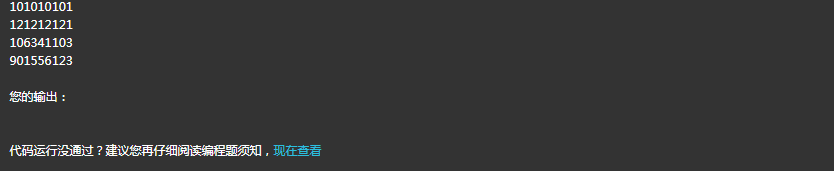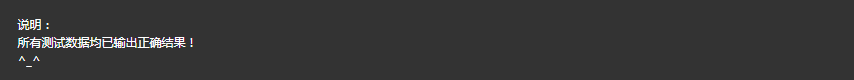病毒

题目描述


 输入输入中有多组测试数据。每组测试数据在单独的一行中，为整数n(1< =n < =10^9)。 样例输入1020 输出对每组测试数据，在单独的行中输出问题的答案。 样例输出23 时间限制C/C++语言：1000MS其它语言：3000MS 内存限制C/C++语言：65536KB其它语言：589824KB
#include<iostream> #include <iomanip> #include<math.h> using namespace std; int main() { int N; int m; while(cin >> N) { int num=0; for(int i=1;i<=N;i++) { for(m=i;m!=0;m=m/10) { if((m%10)>1) break; } if(m==0) { num++; } } cout<<num; } return 0; }#include<iostream> #include<iomanip> #include<math.h> using namespace std; int main() { char N; while(cin >> N) { int num=1; for(int i=0;N[i];i++) { if(N[i]>'1') { for(int j=i;N[j];j++) { N[j]='1'; } } } for(int i=1;N[i];i++) { num=num*2; if(N[i]=='1') num++; } cout<<num<<endl; } return 0; }